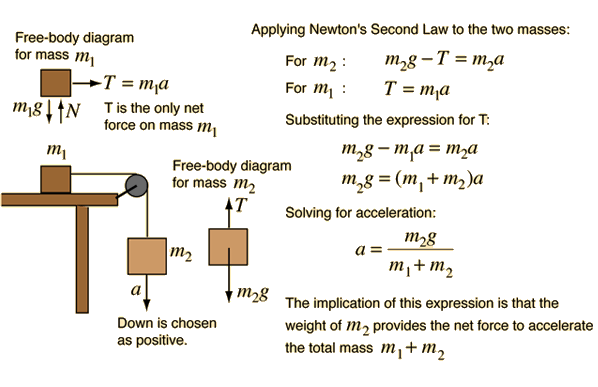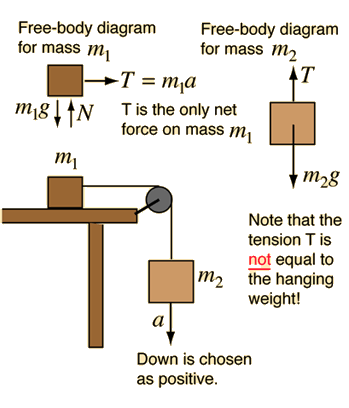# Horizontal PulleyIndex

Newton's laws

Standard mechanics problems

 HyperPhysics***** Mechanics R Nave
Go Back

# Horizontal PulleyApplication of Newton's second law to a horizontal pulley.
Given a mass of kg placed on a horizontal table. It is attached by a rope over a pulley to a mass of kg which hangs vertically. Taking downward as the positive direction for the hanging mass, the acceleration will be

### Acceleration =m/s²

With this acceleration, the tension in the rope will be
T= Newtons compared to the weight W = Newtons for the hanging mass.